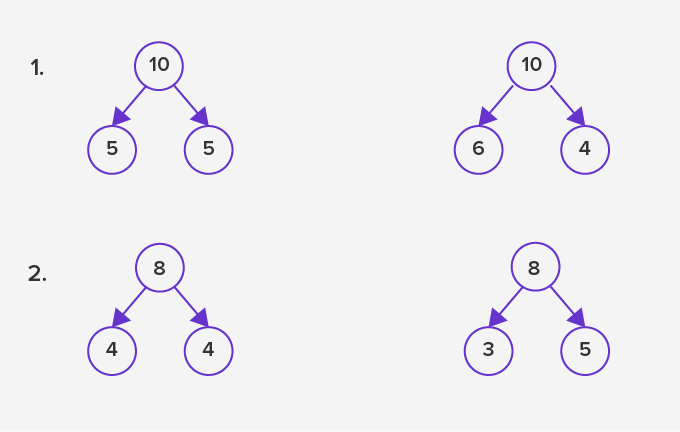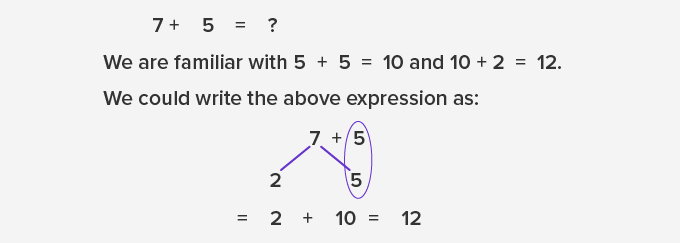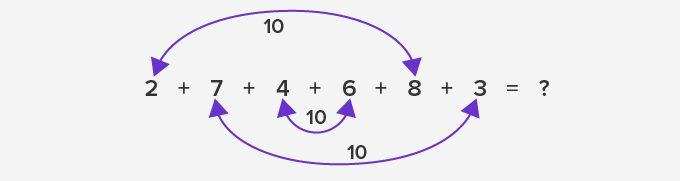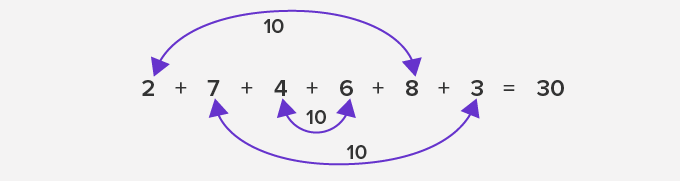Number Bonds - Definition with Examples

The Complete K-5 Math Learning Program Built for Your Child

• 30 Million Kids

Loved by kids and parent worldwide

• 50,000 Schools

Trusted by teachers across schools

• Comprehensive Curriculum

Aligned to Common Core

Number Bonds

A number bond is a simple addition of two numbers that add up to give the sum. Using number bonds, one can instantly tell the answer without the need for the actual calculation.In the example given we can see that when we see a number bond, we instantly know the answer, without having to calculate.

Number bonds are also considered as pictures that show the bond between parts and whole.

It is very useful while learning addition and subtraction in basic arithmetic. For example:In the example above we broke the number 7 into two parts. The value of the first part is 2 and the value of the second part is 5. And while we are familiar with, 5 + 5 = 10

It becomes easier to find that 10 + 2 = 12

Number bonds help us to understand that a whole number is made up of parts. These parts could be in different proportions.

For example, a few of the number bonds of 12 are:Number bonds are also helpful when we need to add many different numbers. In such case, grouping of similar numbers helps to make addition easy.

For example:

2 + 7 + 4 + 6 + 8 + 3 = ?

In this case, if we know the number bonds for 10, we can easily combine the numbers that give 10 and make our calculations simpler and faster.So here, we have 2 + 8 = 10; 7 + 3 = 10 and 4 + 6 = 10. So, we get the sum as 10 + 10 + 10 = 30Through number bonds, we can also solve the problems of subtraction.

Example: 10 –  ____  = 8

Here, we know that 10 is the whole number and 8 is one of the numbers from the pair of number bonds.  The other number that combines with 8 to give 10 is 2.

So, 10 – 2 = 8Fun fact: If we know the 3 numbers of a number bond, we can make 2 addition and 2 subtraction sentences with those 3 numbers. For example:Won Numerous Awards & Honors